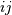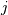# scipy.cluster.hierarchy.cophenet¶

scipy.cluster.hierarchy.cophenet(Z, Y=None)[source]

Calculates the cophenetic distances between each observation in the hierarchical clustering defined by the linkage Z.

Suppose p and q are original observations in disjoint clusters s and t, respectively and s and t are joined by a direct parent cluster u. The cophenetic distance between observations i and j is simply the distance between clusters s and t.

Parameters: Z : ndarray The hierarchical clustering encoded as an array (see linkage function). Y : ndarray (optional) Calculates the cophenetic correlation coefficient c of a hierarchical clustering defined by the linkage matrix Z of a set ofobservations indimensions. Y is the condensed distance matrix from which Z was generated. c : ndarray The cophentic correlation distance (if y is passed). d : ndarray The cophenetic distance matrix in condensed form. Theth entry is the cophenetic distance between original observationsand.

#### Previous topic

scipy.cluster.hierarchy.ward

#### Next topic

scipy.cluster.hierarchy.from_mlab_linkage